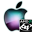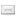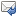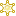ATOMS : Plotting library details

# Plotting library

"Matlab-like" Plotting library for Scilab
Details
Version
0.22
A more recent valid version with binaries for Scilab 5.2 exists: 0.42
Author
Stéphane MOTTELET
Owner Organization
Université de Technologie de Compiègne
Maintainers
Stéphane MOTTELET
Pierre MARECHAL
Category
Creation Date
September 24, 2010
Source created on
Scilab 5.2.x
Binaries available on
Scilab 5.2.x:Windows 64-bitWindows 32-bitLinux 64-bitLinux 32-bitmacOS
Install command
`--> atomsInstall("plotlib")`
Report a bugDescription
```            This library is supposed to help you to make plots as if you were using Matlab.

Plotlib version 0.22 is a dependency for XMLlab 1.75

By default all plotlib function names are defined with a leading underscore, for
example

_gcf, _gca, _plot, _surf, ...

in order to differentiate these plotlib functions from the original scilab
functions having the same name. If you want to use the classical names without
underscores, which will break some native Scilab graphic functions, just add the
line

plotlibmode

in your .scilab file, which can be edited by calling

editor SCIHOME/.scilab
```Files (2)Source code archive
```
```OS-independent binary for Scilab 5.2.x
```Binary version
Automatically generated by the ATOMS compilation chain

```News (0)Comments (3)Comment from GM Culp -- February 17, 2011, 01:17:23 AM
```Good evening!

I am new to SciLab and just installed your toolbox.  I have been experimenting with your
demos which are quite impressive.  I would like to use your 3d functions to plot color
space functions.

For example, here are the equations for the LMS model where r,g,b are values ranging from
0-1:

l=((17.8824).*r)+((43.5161).*g)+((4.11935).*b);
m=((3.45565).*r)+((27.1554).*g)+((3.86714).*b);
s=((0.299566).*r)+((0.184309).*g)+((1.46709).*b);

I see you use meshgrid(x,y) in one of your examples.  I attempted to use meshgrid(x,y,z)

code:

[r,g,b]=meshgrid(linspace(0,1,11),linspace(0,1,11),linspace(0,1,11));

_mesh(((17.8824).*r)+((43.5161).*g)+((4.11935).*b),((3.45565).*r)+((27.1554).*g)+((3.86714).*b),((0.299566).*r)+((0.184309).*g)+((1.46709).*b),'edgecolor','blue');

resulted in the following:
-->exec('C:\Program Files\scilab-5.3.0\test5.sce', -1)
!--error 10000
mesh : argument 1 must be a matrix or function
at line       7 of function _error called by :
at line      19 of function _checkTypeAndDimensions called by :
at line      47 of function _mainPlot called by :
at line      15 of function _mesh called by :
99566).*r)+((0.184309).*g)+((1.46709).*b),'edgecolor','blue')
at line       3 of exec file called by :
exec('C:\Program Files\scilab-5.3.0\test5.sce', -1)

I what I am attempting to do possible in SciLab?  If so, what am I doing wrong?

Thank you!

Gretchen```Answer from Stéphane MOTTELET-- February 17, 2011, 08:48:01 AM
```> Good evening!
>
> I am new to SciLab and just installed your toolbox.  I have been experimenting with
> your
> demos which are quite impressive.  I would like to use your 3d functions to plot color
> space functions.
>
> For example, here are the equations for the LMS model where r,g,b are values ranging
> from 0-1:
>
> l=((17.8824).*r)+((43.5161).*g)+((4.11935).*b);
> m=((3.45565).*r)+((27.1554).*g)+((3.86714).*b);
> s=((0.299566).*r)+((0.184309).*g)+((1.46709).*b);
>
> I see you use meshgrid(x,y) in one of your examples.  I attempted to use
> meshgrid(x,y,z)
>
> code:
>
> [r,g,b]=meshgrid(linspace(0,1,11),linspace(0,1,11),linspace(0,1,11));
>

>
_mesh(((17.8824).*r)+((43.5161).*g)+((4.11935).*b),((3.45565).*r)+((27.1554).*g)+((3.86714).*b),((0.299566).*r)+((0.184309).*g)+((1.46709).*b),'edgecolor','blue');
>
> resulted in the following:
> -->exec('C:\Program Files\scilab-5.3.0\test5.sce', -1)
>  !--error 10000
> mesh : argument 1 must be a matrix or function
> at line       7 of function _error called by :
> at line      19 of function _checkTypeAndDimensions called by :
> at line      47 of function _mainPlot called by :
> at line      15 of function _mesh called by :
> 99566).*r)+((0.184309).*g)+((1.46709).*b),'edgecolor','blue')
> at line       3 of exec file called by :
> exec('C:\Program Files\scilab-5.3.0\test5.sce', -1)
>
> I what I am attempting to do possible in SciLab?  If so, what am I doing wrong?
>
> Thank you!
>
> Gretchen

Hi Gretchen,

you cannot use hypermatrices (r,g,b are of this type in your example) with mesh or surf,
or any 3D surface plotting function. Do you have an example image of what you are trying
to represent ?

S.
```Comment from Stéphane MOTTELET-- February 17, 2011, 08:47:06 AM
```Hi Gretchen,

you cannot use hypermatrices (r,g,b are of this type in your example) with mesh or surf,
or any 3D surface plotting function. Do you have an example image of what you are trying
to represent ?

S.```Comment from GM Culp -- February 17, 2011, 07:59:06 PM
```Good afternoon, Stéphane!

Here is a link to a graph that plots CIELab space:
http://www.gamutvision.com/docs/images/GV_printest_Lab3D_2200PremLuster.jpg

RGB space is a cube but other spaces have different geometries (LMS, for example, looks
like a book).  I would use these graphs not only to illustrate what color blind users
don't see (for example, the red and green corners of the RGB cube would be missing for
someone with red/green color blindness) but also colors that are most different (furthest
apart in the color space) for creating accessible images and maps.

I have also tried running different colors through the color space equation and then
plotting them as points.  It works OK but I would love to stretch a surface over the point
cloud so the geometry is easier to see.

Thank you so much for responding to my query!

Gretchen```Leave a comment
Login withEmail notifications